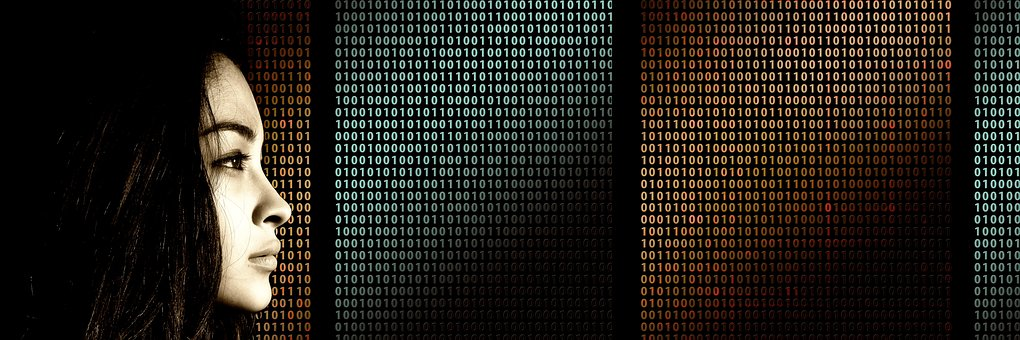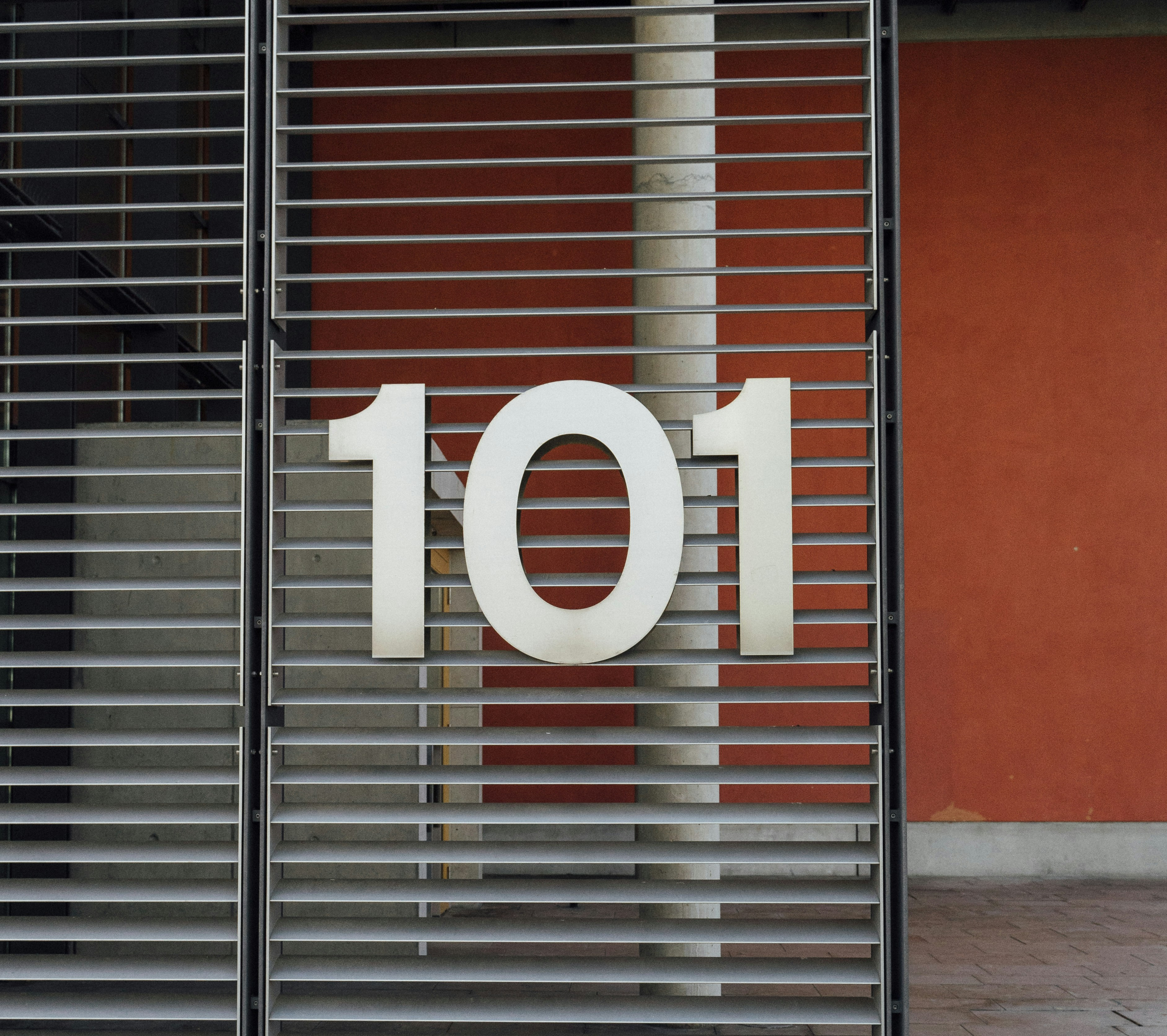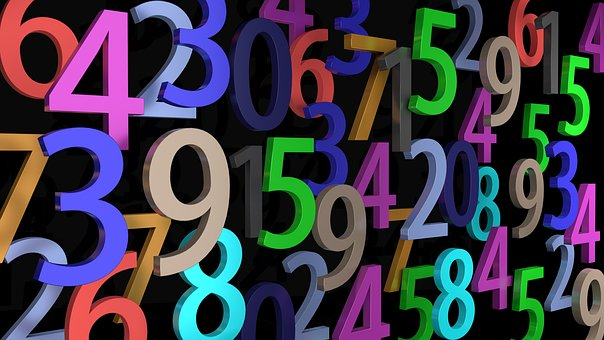### Hex:

It’s indeed necessary to convert the value from one number system to some other number system. With this, we will be in a position to say when the two different values are going to reflect a similar number. When converting decimal to binary, we convert decimal number system to binary number system using a simple method. In this article, we will address the conversion of base 10 numbers to base 2 numbers by some examples.## Decimal Numbers

In algebra, numbers can be divided into various groups, including actual numbers, natural numbers, whole numbers, rational numbers, and many more. There are decimal numbers among them. It should be the normal type of representation of integer and non-integer numbers.

## Binary Numbers

In mathematics, the binary number systems are the positional numeral system that uses 2 as the base and thus needs only two different symbols for its digits, 0 and 1, instead of the normal 10 different symbols required in the decimal system. The significance of the binary system to the philosophy of information and computer technology stems primarily from the compact and secure way in which it is used.

## Binary, The Numbering System of Base 2

In the decimal number system, we found that ten numbers were used to represent numbers from zero to nine.

Binary uses only two numbers 0 and 1. Place holders in binaries each have a power value of 2. So the first place is 2^0 = 1, the second place is 2^1 = 2, the third place is 2^2 = 4, the fourth place is 2^3 = 8 and so on.

In binary, we count 0, 1 and then, because there is no number for two, we pass to the next holder, so two is written as 10 binary. This is almost the same as when we get to 10 decimal places and have to write it to 10 because there is no number for 10.

## Decimal, The Numbering System of Base 10

The decimal, also classified as the denary or base 10 numbering scheme, is which we use for counting in daily life. The reason that there are 10 symbols is more than likely that we have 10 fingers.

We use ten various symbols or numbers to represent numbers from zero to nine.

These numbers are 0, 1, 2, 3, 4, 5, 6, 7, 8 and 9.

We don’t have a numeral to represent this value when we get to the number 10, so it’s written as it is.

The concept is by using a new place holder for each power of 10 to make up any number that we want.

So 134 mean one hundred, three tens and four, even though we’re only interpreting and reading it as one hundred and thirty-four.

## Most Significant Bit (MSB) and Least Significant Bit (LSB)

For such a binary number, most significant bit (MSB) is the digit to the left of the number, and indeed the least significant bit (LSB) is the digit to the right.

## Decimal Conversion to Binary Conversion

The decimal number has base 10; the binary number has base 2. In decimal to binary conversion, the number base often shifts, i.e. from base 10 to base 2. Both decimal numbers have their corresponding binary numbers. These binary numbers are primarily used in computer programs where they are used for computer or coding purposes. That is though computers realize the binary digit language, o and 1.

Thus, when we send the input to the computer system in decimal form, it converts it to binary digits, takes the following operations, and returns the information to decimal form again.

## Steps to Convert Decimal to Binary

If you’re not using a calculator by side, you can simply convert a decimal number to a binary by using rest process. This means splitting the number by 2 recursively until you are left with 0, thus taking note of each remainder.

1. Please write down the decimal number.
2. Divide the number by two.
3. Write down the result.
4. Write the rest of it on the right hand page. This is going to be 0 or 1.
5. Divide the product of the division by 2 and note down the remainder.
6. Start to divide and write down the remainders when the product of the division is 0.
7. The most significant bit (MSB) has been at the bottom of the rest column and the least significant bit (LSB) would be at the top.

## Decimal Numbers to Binary Numbers Table

For convert numbers from decimal to binary number method, note the decimal to binary table to find solutions effectively with an appropriate result. The decimal to binary conversion of up to 20 numbers is given below for guidance.

 DECIMAL NUMBERS BINARY NUMBERS 1 1 2 10 3 11 4 100 5 101 6 110 7 111 8 1000 9 1001 10 1010 11 1011 12 1100 13 1101 14 1110 15 1111 16 10000 17 10001 18 10010 19 10011 20 10100## Methods of Conversion

Below we go through two methods of conversion of decimal to binary.

We’re going to turn the decimal number 57 into a binary number. The techniques below may be extended to any positive integer decimal number.

### Method 1: Declining Powers of Two and Subtraction

Before we do some calculation, we’re going to write down the powers of 2. We’re going to use this example to make it easier to follow. We only need to write down the powers of 2 before we reach the decimal number to be converted. However, in our case, we’re going to write up to 256.

2^0=1

2^1=2

2^2=4

2^3=8

2^4=16

2^5=32

2^6=64

2^7=128

2^8=256

Next, we write down the decimal number as a percentage of the powers of 2.

After looking at the power of 2 tables above, we can extract the maximum power of 2 which is less than our decimal number (57). It’s 32 in our situation. So we’re going to write 57 for the number of 32 and 25. As we continue with 25, so we write it as a sum of the powers of 2. We’ll eventually get:

57=32+25

=32+16+9

=32+16+8+1

First, we’re going to multiply with 1 per power of 2. The number remains the same but is written closer to the binary extraction:

57=1.32+1.16+1.8+1.1

We write the similar expression, except with the power of 2:

57=1.2^5+1.2^4+1.2^3+1.2^0

Today we add the missing powers of 2 to the sum, but multiplied by 0 (zero) to hold the same result:

57=1.2^5+1.2^4+1.2^3+0.2^2+0.2^1+1.2^0

From either the expression above, we obtain the coefficients of power of 2, which is the binary number:

111001

Finally, the representation in binary format of 57 is as follows:

57=111001

### Method 2: Small Division by Two with the Remainder

The second form is simpler and probably easier to recall than the first.

Let’s start by dividing our decimal number 57 by 2. The product of the division is 28, with the remainder being 1. We keep the rest in mind and begin to divide 28 by 2. The outcome is 14, with the remaining 0. We shall continue with the division until the product of the division is equal to 1:

57÷2(1)

28÷2(0)

14÷2(0)

7÷2(0)

3÷2(1)

1÷2(1)

By removing all the remains of the divisions, we should get binary representation of the decimal number. The remainder of the first division is the first bit (from right).

After putting all the remnants next to each other, we get the binary representation of the decimal number:

111001

Finally, the representation in binary format of 57 is as follows:

57=111001

Obviously, we’ve got the identical result as Method 1.

Since we need to display the decimal number on 8 bits, we would only have to fill the left side of the binary representation with 0 till we have a total of 8 bits:

57=01111001

## Indicating the Number of Base

The binary number 1011011 can be written as 1011011 with base 2 to show the foundation directly. Similarly, 54 Base 10 can be written 54 with base 10. However, a subscription is always omitted to prevent unnecessary detail when the meaning is understood. Usually subscriptions are only used in the explanatory text or comments in the code to prevent confusion when several numbers of different bases are used together.

## The Other Bases Aside from 2 and 10

Base 16 or hexadecimal (hex for simple) is a standard used for programming computer systems. It uses 16 symbols, describing 10, 11, 12, 13, 14 and 15 decimal letters A, B, C, D, E and F collectively.## Conversion of Decimal Numbers to Binary Numbers

There are several types of decimals in this conversion that we use to convert to a binary number scheme.

### Integer Decimal Numbers for Binary

Let’s dividing the total number by 2 and only take the remainder, if the division is done then only take the remainder, which gives the binary number.

Suppose that we are converting the decimal number 87 with base 10. We divide 87 by 2 and get 43 as a quotient and 1 as the rest.

The probability of remaining (87)10 = (1010111)2 is just 1 and 0. As a consequence, the number is counted from the last remainder. For example, 1→0 →1→ 0→ 1→ 1→  1. This is how decimal to binary conversions are achieved.

### Fraction Decimal Numbers for Binary

The successive multiplication is performed in this case. The number to be modified is multiplied by the base or radix of the binary number, which is 2. The whole or part of the product is extracted and the same procedure is repeated until we can get an integer.

0.95×2=1.90———–1

0.90×2=1.80———–1

0.80×2=1.60———–1

0.60×2=1.20———–1

0.20×2=0.40———–1

0.40×2=0.80———–0

Since we do not get an integer value after a continuous multiplication, we can calculate the value to be 111110.

### Negative Decimal to Binary

In the case of a negative number, we can go to the 2 supplementary representation of a signed number.

Example:  9 = 0000 1001

Complement of 1 = 1111 0110

Adding 1 we get = 11110111 which is a complement of 2 and represented by (-9).

## Why Don’t All Fractions can be Countable expressed in binaries

The assumption that certain fractions finitely represented in the decimal system cannot be finitely represented in the binary system means a great deal too many developers. But this is precisely the mystery that lies at the root of the apparently odd result of adding 0.1 to 0.2. So what decides whether a fraction can be finitely expressed in a numerical system?

Well, in order for a number to be finitely represented, the denominator should have a fraction of the power of the system base. For example, for the base-10 scheme, the denominator should have a power of 10, which is why we can finally have a decimal value of 0.625:

(625/10^3)=0.625

And can’t represent 1/3 in the end

1/3=0.333…

The same applies to the Base-2 system:

(625/1000)=(5/8)=(5/2^3)

Though if we search 0.1, the denominator is 10 and it’s not 2 and 0.1 is going to be an infinite fraction in the binary system. Here’s how it using the algorithm we’ve learned above:

0.1 x 2 = 0 + 0.2

0.2 x 2 = 0 + 0.4

0.4 x 2 = 0 + 0.8

0.8 x 2 = 1 + 0.6

0.6 x 2 = 1 + 0.2

0.2 x 2 = 0 + 0.4

0.4 x 2 = 0 + 0.8

We can continue to do something like that infinitely, now let’s write it down as a continuous periodic fraction:

Below you are given with some most frequently asked questions related with conversion of decimal to binary.

### How am I going to convert decimal to binary?

The easiest way to convert decimal to binary number equivalents is to write down the decimal number and continuously divide-by-2 (two) to give the result and the remainder either a “1” or a “0” till the final result reaches zero.

### What do 01011 mean by binary?

In Binary the representation is:  010111 = 11

### How are you going to find binary numbers?

To measure the number value of a binary number, calculate the value of all 1s to the eight character number for each position.

## Conclusion

Learners can understand how to convert decimal to binary. They’re going to hear about the power of 1’s and 0’s. Finally, the instructor will ask the students the Cisco Binary Game and encourage them to play to improve and strengthen their skills.